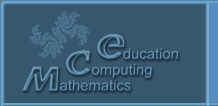Русский
 ! Вопросы и ответы

# Abstracts

## Economical tasks in the course of basic mathematical subjects

Gubareva E.A., Parshikova G.Y.

State university of management Department of mathematics Russia, 109 542, Moscow, Ryazan prospectus, d.99. Tel. (495) 371-70-88, fax: (495) 371-70-88, E-mail: gubel@inbox.ru

1 pp. (accepted)

For all basic mathematical subjects of economical institutes it is very important for them to be practical when fundamental results of the theory are illustrated by economical tasks. For mastering methods of solution of these tasks the educational supplies “Tasks and exercises on economy and management in basic mathematical subjects”  is made up. The brochure is composed as a complementation to the textbook by V.V. Lebedev  and includes four themes from the programs of subjects “Mathematics” and “Mathematical analysis”: introduction in mathematical analysis, differential calculus of functions of one variable, differential calculus of functions of several variables, common differential equations.

Taking into considerations features of modern students the brochure is built on the principal of “do with us, do like us”. About 30 basic economical tasks are scrutinized and for each subjects 10 similar tasks are suggested to be solved on one’s own. These tasks are included in the final test.

What does a Bachelor have to know? On the first subject – to find a break-even point of a one-product firm, point of equilibrium in the market, build a budget set of a consumer of two projects. On the second subject – to build graphics of the functions: full, marginal and average cost of an one-product firm; revenue, average and marginal revenue; to find the coefficient of elasticity of a function of product demand, optimal output of a one-product firm. On the third subject – to build set of production opportunities of the firm, isoquants of different production functions, to find marginal productivities of products, marginal rate of substitution of one product by another, elasticity coefficients of production function by labor and capital, to solve optimization tasks of models of the production, two-product firm, consumer’s behavior. On the fourth subjects – to solve simplest dynamic models.

References.

1. Y.A. Aganin, M.V. Bukhtoyarova, E.A., E.A. Gubareva, V.V. Lebedev, G.Y. Parshikova. “Tasks and exercises on economy and management in basic mathematical subjects” under general edition by V.V. Lebedev. − M.: GUU, 2013.

2. Lebedev V.V. Mathematics in economics and management. – M.: NТV – “Dizain”, 2004.

© 2004 Designed by Lyceum of Informational Technologies №1533